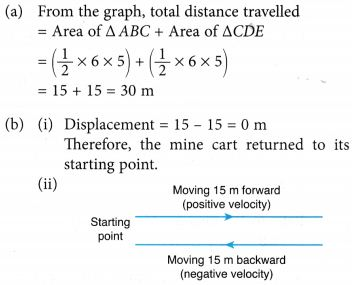## What is Speed

• When we say a body moves fast (or slow) we refer to its speed.
• The speed of an object is defined as the distance travelled by it in unit time.
.The SI unit of speed is metre per second (m/s).
• Therefore, an object that moves faster covers more distance in a second (unit time) as compared to an object that moves slower.

### Different Units of Speed

Depending on the need and context, speed is measured in different units. As you already know, the SI unit of speed is metre per second. Another commonly used unit is kilometre per hour (km/h). When we talk about speed of aeroplanes and cars, it would be more appropriate to use km/h than m/s. Let us see how the speed of the school bus (given in Example 1) can be expressed in km/h.
Speed In km/h
Distance travelled by the bus = 7200 m
Distance travelled in kilometres = 7200 ÷ 1000 = 7.2km
Time taken = 1800 s
Time in hours = 1800 ÷ 3600 = O.5h
Therefore, speed of the bus = 7.2 ÷ 0.5 = 14.4 km/h

Speed in m/s can be easily converted to km/h by dividing the answer in m/s by 5/18 or multiplying by 18/5. Do you know how we got this?
1 km = 1000 m
1 h = 3600 s
1 km/h = 1000 m/3600 s
= 5/18 m/s

When a car (or any other vehicle) is moving, it is interesting to find out how fast it is moving. Have you seen a meter fitted inside a vehicle? In a car, the meter is fitted on the dashboard, and in scooters or motorcycles on the top. This is used to display the speed and distance travelled by the vehicle. The instrument/meter measuring the speed of the vehicle is called a speedometer. The distance travelled by the vehicle is given by an instrument called odometer. Both of them are usually fitted together. These instruments will give the reading of speed and the distance travelled at the same time.

### Calculation of Speed

Shreya, Ronald, Sabina, and Kirit participated in a race. They took 20s, 22s, 25s, and 28s, respectively, to reach the finishing line. Who do you think ran the fastest and who ran the slowest?
We can calculate the speed of an object if we know the distance it travels in a given amount of time. Let us see how this is done.In the above example, let us say it was a 100 m race. Shreya ran the race in 20 s. What is Shreya’s speed? As speed is the distance travelled in unit time, Shreya’s speed can be calculated by dividing the distance she ran by the time she took to cover that distance.
Therefore, Shreya’s speed is
$$\frac{\text{Distance travelled}}{\text{Time taken to travel the distance}}=\frac{\text{100 m}}{\text{20 s}}=5~\text{m/s}$$
Similarly, calculate the speed of Ronald, Sabina, and Kirit.
Let us consider one more example.

Example 1:    A school bus covers a distance of 7200 m in 1800 s. Calculate its speed.
Solution:    We know that speed is given by the distance travelled divided by the time taken.
Given: Distance travelled by the bus = 7200 m
Time taken = 1800 s
$$\text{Speed}=\frac{\text{Distance travelled}}{\text{Time taken }}$$
$$=\frac{\text{7200 m}}{\text{1800 s}}=4~\text{m/s}$$

In practice, very few objects travel at a constant speed for long. Your school bus may speed up and slow down many times on the way to school. To calculate the speed in such cases, we use the concept of average speed. Average speed is defined as the total distance travelled divided by the total time taken to travel that distance.

## Difference Between Speed And Velocity

• The ‘distance’ travelled by a body in unit time interval is called its speed. When the position of a body changes in particular direction, then speed is denoted by ‘velocity’. i.e. the rate of change of displacement of a body is called its Velocity.
• Speed is a scalar quantity while velocity is a vector quantity.
• $$\text{Speed}=\frac{\text{distance}}{\text{time}}$$
• $$\text{Velocity}=\frac{\text{displacement}}{\text{time}}$$
• Unit: In M.K.S. system = ms-1
In C.G.S. system = cm/s
• If time distance graph is given then speed can be given by the slope of the line, at given time
$$\text{V}=\frac{\text{ }\!\!\Delta\!\!\text{ s}}{\text{ }\!\!\Delta\!\!\text{ t}}=\text{Slope}$$• The area of velocity time graph gives displacement travelled.

### Types of speed

(a) Average and Instantaneous speed
Average speed: It is obtained by dividing the total distance travelled by the total time interval. i.e.
$$\text{Average speed}=\frac{\text{total}\,\,\text{distance}}{\text{total}\,\,\text{time}}$$
$$\text{Average}\,\text{velocity}=\frac{\text{displacement}}{\text{total}\,\,\text{time}}$$

• Average speed is a scalar, while average velocity is a vector.
• For a moving body average speed can never be –ve or zero (unless t → ∞), while average velocity can be i.e
$${{v}_{av~}}>0\text{ while }\overset{\to }{\mathop{{{v}_{av}}}}\,>=or<\text{ }0$$
• In general average speed is not equal to magnitude of average velocity. However it can be so if the motion is along a straight line without change in direction
• If a particle travels distances L1, L2, L3 at speeds v1, v2, v3 etc respectively, then
$${{v}_{av~}}=\frac{\Delta s}{\Delta t}=\frac{{{L}_{1}}+{{L}_{2}}+…..+{{L}_{n}}}{\frac{{{L}_{1}}}{{{v}_{1}}}+\frac{{{L}_{2}}}{{{v}_{2}}}+….+\frac{{{L}_{n}}}{{{v}_{n}}}}=\frac{\sum{Li}}{\sum{\frac{{{L}_{i}}}{{{v}_{i}}}}}$$
• If a particle travels at speeds v1, v2 etc for intervals t1, t2 etc respectively, then
$${{v}_{av~}}=\frac{{{v}_{1}}{{t}_{1}}+{{v}_{2}}{{t}_{2}}+….}{{{t}_{1}}+{{t}_{2}}+….}=\frac{\sum{{{v}_{1}}{{t}_{1}}}}{\sum{{{t}_{1}}}}$$

Instantaneous speed: The speed of a body at a particular instant of time is called its instantaneous speed.
$$=\underset{\Delta t\to 0}{\mathop{\lim }}\,\,\frac{\Delta s}{\Delta t}=\frac{ds}{dt}$$

(b) Uniform and Non uniform speed
Uniform speed: If an object covers equal distance in equal interval of time, then time speed graph of an object is a straight line parallel to time axis then body is moving with a uniform speed.
Non-uniform speed: If the speed of a body is changing with respect to time it is moving with a non-uniform speed.

## Speed And Velocity Example Problems With Solutions

Example 1. The distance between two points A and B is 100 m. A person moves from A to B with a speed of 20 m/s and from B to A with a speed of 25 m/s. Calculate average speed and average velocity.
Solution:    (i) Distance from A to B = 100 m
Distance from B to A = 100 m
Thus, total distance = 200 m
Time taken to move from A to B, is given by
$${{t}_{1}}=\frac{\text{distance}}{\text{velocity}}=\frac{100}{20}=5\text{ seconds}$$
Time taken from B to A, is given by
$${{t}_{2}}=\frac{\text{distance}}{\text{velocity}}=\frac{100}{25}=4\text{ seconds}$$
Total time taken = t1 + t2 = 5 + 4 = 9 sec.
∴ Average speed of the person
$$=\frac{\text{Total}\,\text{dis}\,\text{tan}\,\text{cecovered}}{\text{Total}\,\text{time}\,\text{taken}}=\frac{200}{9}=22.2\text{ m/s}$$
(ii) Since person comes back to initial position A, displacement will be zero, resulting zero average velocity.

Example 2. A car moves with a speed of 40 km/hr for first hour, then with a speed of 60 km/hr for next $$1\frac{1}{2}$$ half hour and finally with a speed of 30 km/hr for next hours. Calculate the average speed of the car.
Solution:    Distance travelled in first hour, is given by
s1 = speed × time = 40 km/hr × 1 hr = 40 km
Distance travelled in next half an hour, is given by
s2 = speed × time = 60 km/hr × $$\frac { 1 }{ 2 }$$ hr = 30 km
Distance travelled in last $$1\frac{1}{2}$$ hours, is given by
s3 = speed × time = 30 km/hr × $$\frac { 3 }{ 2 }$$ hr = 45 km
Thus, total distance travelled = s1 + s2 + s3
= 40 + 30 + 45 = 115 km
Total time taken = 1 + $$\frac { 1 }{ 2 }$$ + $$1\frac{1}{2}$$ = 3 hours
Average speed = $$\frac { Total distance covered }{ Total time taken }$$ = $$\frac { 115km }{ 3hrs }$$
= 38.33 km/hr

Example 3. Figure shows time distance graph of an object. Calculate the following :
(i) Which part of the graph shows that the body is at rest ?
(ii) Average speed in first 10 s.
(iii) Speeds in different parts of motion.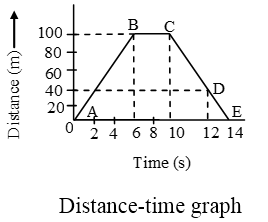Solution:    (i) The part BC shows that the body is at rest.
(ii) In first 10 seconds, distance travelled = 100m
$$\text{Average speed}=\frac{\text{total}\,\,\text{distance}}{\text{total}\,\,\text{time}}$$
$$=\frac{100}{10}=10\text{ m/s}$$
(iii) Speed of the object in part AB is given by slope = 100/6 = $$\frac { 50 }{ 3 }$$ m/s
Speed of object in part BC = 0 m/s
Speed of the object in part CD
$$=\frac{100-40}{12-10}=\frac{60}{2}=30~\text{m/s}$$
Speed of object in part DE
$$=\frac{40-0}{14-12}=\frac{40}{2}=30~\text{m/s}$$

Example 4. Time-velocity graph of a particle is shown in Figure. Calculate the distance travelled in first seconds.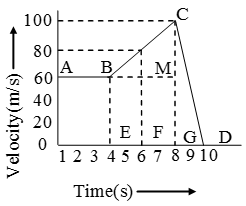Solution:    Distance travelled in first 8s is given by area OABCG
= area of rectangle OAMG + area of triangle BMC
= 8 × 60 + $$\frac { 1 }{ 2 }$$ × 4 × 40
= 480 + 80 = 560 m.

Example 5. A cow walked along a curved path from P to Q, which is 70 m away from P. Q lies to the south-west of P. The distance travelled by the cow is 240 m and the time taken is 160 s.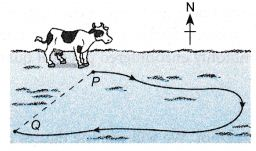Calculate the
(a) average speed,
(b) average velocity,
of the cow moving from P to Q.
Solution:
Total distance travelled = 240 m
Displacement = 70 m
Time taken = 160 s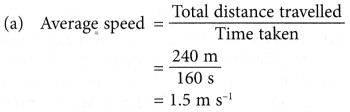## What is Motion

When a body does not change its position with time, we can say that the body is at rest, while if a body changes its position with time, it is said to be in motion.

Analysing Linear Motion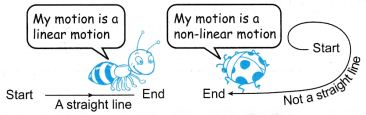• Linear motion is motion in a straight line.
• Non-linear motion is motion that is not in a straight line.
• When analysing linear and non-linear motion, distance, displacement, speed, velocity, acceleration and deceleration are some commonly encountered physical quantities.

Terms Used To Define Motion

An object is said to be a point object if it changes its position by distances which are much greater than its size. A point or some stationary object with respect to which a body continuously changes its position in the state of motion is known as origin or reference point.

When we say an object is moving, it is always with respect to another object. For example, when we say a vehicle is moving on a road, it is moving with respect to the trees and poles on the road, which are ‘not moving’. When you say you are at rest or not moving while reading a book, it is with respect to your chair or your desk. When an object moves, its position (with respect to another object) changes with time. Let us take the example of a car moving away from a building.When the car is at position A, the distance between the car and the building is very small. As the car moves away from the building (Position B), its distance from the building increases. Another way of saying this is that when the car moves, its position with respect to the building changes with time. An object is said to be in motion if its position (with respect to another object) changes with time.

### Types Of Motion

There are different types of motion: translational, rotational, periodic, and non periodic motion.

Translational Motion
A type of motion in which all parts of an object move the same distance in a given time is called translational motion. Examples are vehicles moving on a road, a child going down a bird flying in the sky. Translational motion can be of two types, rectilinear and curvilinear. Table shows the differences between rectilinear and curvilinear motions.

 Rectilinear motion Curvilinear motion 1. When an object in translational motion moves in a straight line, it is said to be in rectilinear motion. 1. When an object in translational motion moves along a curved path, it is said to be in curvilinear motion. 2. Examples are a car moving on a straight road and a train moving on a straight track. 2. Examples are a stone thrown up in the air at an angle and a car taking a turn.Examples of translational motion: a child going down a slide.

Rotational Motion
When an object moves about an axis and different parts of it move by different distances in a given interval of time, it is said to be in rotational motion. Examples of objects undergoing rotational motion are blades of a rotating fan, merry-go-round, blades of a windmill. When an object undergoes rotational motion, all its parts do not move the same distance in a given interval of time. For example, the outer portion of the blades of a windmill moves much more than the portion closer to the centre.

A merry-go-around shows rotational motion:Periodic Motion
A type of motion that repeats itself after equal intervals of time is called periodic motion. Examples of objects undergoing periodic motion are the to and fro motion of a pendulum, the Earth (rotating on its axis), the hands of a clock, the blades of a rotating electric fan, and the plucked string of a guitar.

Non-periodic Motion
A motion that does not repeat itself at regular intervals or a motion that does not repeat itself at all is called non-periodic motion. Examples of non-periodic motion are a car moving on a road, a bird gliding across the sky, and children playing in a park. In everyday life, we observe more than one type of motion, like

• Birds gliding across the sky (translational and non periodic).
• Rotation of the Earth on its axis (rotational and periodic).According to Directions

1. One dimensional motion is the motion of a particle moving along a straight line.
2. Two dimensional motion A particle moving along a curved path in a plane has
2-dimensional motion.
3. Three dimensional motion Particle moving randomly in space has 3-dimensional motion.

According to State of Motion

1. Uniform Motion: A body is said to be in a state of uniform motion if it travels equal distances in equal intervals of time. If the time distance graph is a straight line the motion is said to be uniform motion.
2. Non-uniform motion: A body has a non-uniform motion if it travels unequal distances in equal intervals of time. Ex. a freely falling body.
Time – distance graph for a body with non-uniform motion is a curved line.

### Analysing Motion GraphsDisplacement- Time graphs:

1. Figure shows a tortoise moving at a slow and steady speed while a hare was sleeping soundly from the time t = 0 to 8 s.
2. We can represent the situation using displacement-time graphs.
(a) The graph in Figure (a) shows the displacement of the tortoise from the starting line from time t = 0 to 8 s.
(b) The graph in Figure (b) shows that throughout the time from t = 0 to 8 s, the hare had a displacement of 0.5 m from the starting line. Throughout the 8 seconds, it did not move at all as it was sleeping.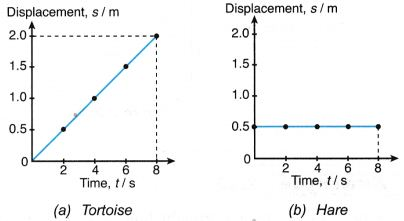3. The gradient of the s-t graph of an object gives the rate of change of displacement, which is the velocity of the object.4. Table illustrates some of the displacement-time graphs.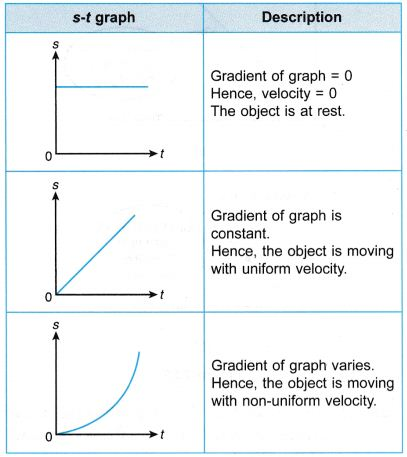The straight line inclined to time axis in s-t graph represents constant velocity.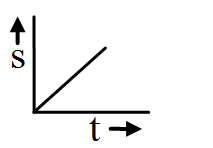In s-t graph the straight line inclined to time axis at angle greater than 90º shows negative velocity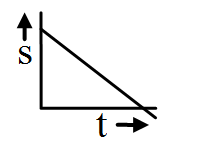Body with accelerated motion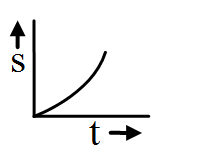Body with decelerated motion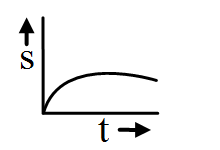Velocity -Time graphs:1. The gradients of the s-t graphs for the tortoise and hare are 0.25 m s-1 and 0 m s-1 respectively.
2. The v-t graphs in Figure represent the situation.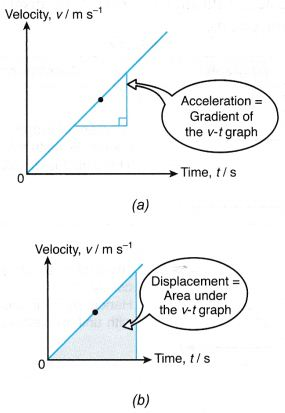(a) The gradient of the v-t graph is the rate of change of velocity which is the acceleration of the object.
(b) The area under the graph gives the displacement of the object.
3. Table illustrates some of the velocity-time graphs.For the body having constant velocity or zero acceleration.The body is moving with constant retardation and its initial velocity is not zero.The body is accelerated and the initial velocity is zero.The body is decelerated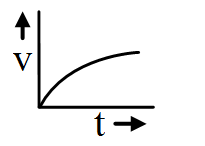Acceleration-Time graph:
Acceleration is constant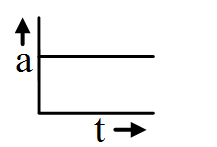Acceleration is increasing and is +ve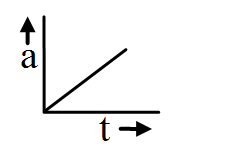Acceleration is decreasing and is –ve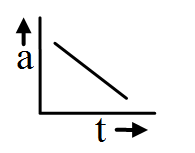Example 1. A boy skated along a straight line passing by two points, P and Q. His time, t and displacement, s from point P is shown in Figure.From the information given, draw the
(a) s-t graph
(b) v-t graph
Solution: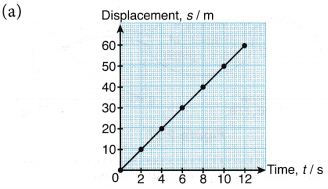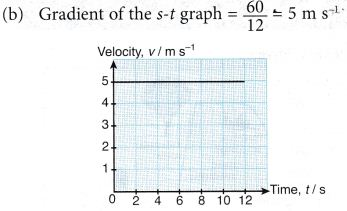Example 2. Azlan cycled along a straight road. The graph in Figure (b) illustrates his motion.(a) Calculate the velocity of Azlan from t = 0 to 10 s.
(b) Describe what happened to Azlan from t = 10 to 20 s.
(c) Calculate the displacement, s of Azlan when t = 25 s.
(d) Where was Azlan when t = 35 s?
Solution:
(a) Refer to part AB of the graph.
Velocity = Gradient of the graph
= 50/10 = 5 m s-1
(b) Refer to part BC of the graph. The gradient of the graph is 0. This means that Azlan was at rest from t = 10 to 20 s.
(c) Refer to point D of the graph. When
t = 25 s, displacement of Azlan, s = 14 m.
(d) Refer to point E of the graph. When
t = 35 s, displacement of Azlan, s = 0 m.
This means that Azlan was back at the starting position.

Example 3. Figure shows a huge crane hoisting a steel beam from the ground. The v-t graph shows the motion of the beam in the first 9 s.(a) Briefly describe the motion of the beam represented by AB, BC, CD and DE.
(b) Calculate the initial and final acceleration of the beam.
(c) Calculate the height of the beam from the ground after 9 seconds.
Solution:Example 4. The v-t graph in Figure shows the motion of a mine cart on a straight railway.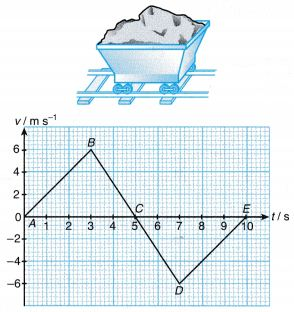(a) What was the total distance travelled by the mine cart?
(b) (i) Where was the mine cart when t = 10 s?